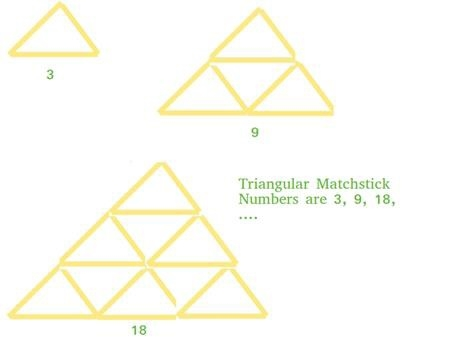# C/C++ Program for the Triangular Matchstick Number?

A triangle that is made by using matchsticks arrange to make an equilateral triangle, this is called the triangular matchstick number. Triangular matchstick number is the number of matchsticks required to make the matchstick triangle.

In this problem, we have the number is the floor of a matchstick pyramid, X. and our task is to write a program to print the total minimum number of matchstick required to form a pyramid of matchsticks of x floors.

Let's look at an example that will make the concept more clear,

Input: 7
Output: 84

## Explanation

This is an extension of triangular numbers. For integer X, the matchstick required will be three times of X-th triangular numbers , i.e., (3*X*(X+1))/2## Example

#include <iostream>
using namespace std;
int main() {
int x=7;
cout<<(3 * x * (x + 1)) / 2;
return 0;
}